## Determining Planet Properties

#### Chapter index in this window —   — Chapter index in separate window -- Please support this website

This material (including images) is copyrighted!. See my copyright notice for fair use practices.

How can you find out what the other planets are like by just observing them carefully from the Earth? Most of the information comes in the form of electromagnetic radiation but we also have little chunks of rock, called meteorites, that give other clues. The image below compares the apparent sizes of the planets. The outer planets are shown at their closest approach to us and the two inner planets are shown at various distances from us (but all are with the same magnification).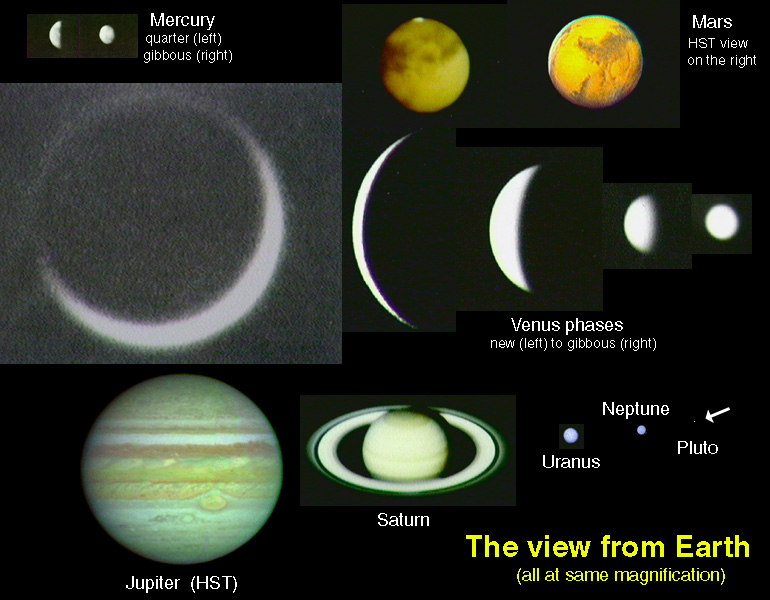Before you can do any sort of comparison of the planets, you need to know how far away they are. Once you know their distances, you can determine basic properties of the planets such as mass, size, and density.

### Distances

Several hundred years ago Copernicus was able to determine approximate distances between the planets through trigonometry. The distances were all found relative to the distance between the Earth and the Sun, the astronomical unit (often abbreviated as "A.U."). Kepler refined these measurements to take into account the elliptical orbits. However, they did not know how large an astronomical unit was.

To establish an absolute distance scale, the actual distance to one of the planets had to be measured. Distances to Venus and Mars were measured from the parallax effect by observers at different parts of the Earth when the planets were closest to the Earth.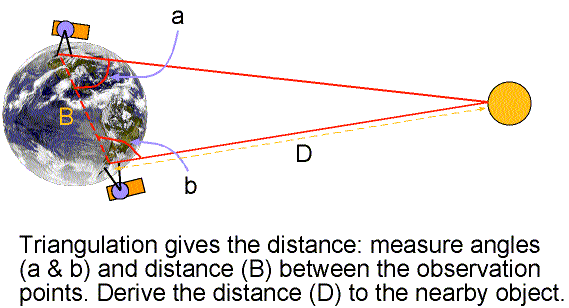Knowing how far apart the observers were from each other and coordinating the observation times, astronomers could determine the distance to a planet. The slight difference in its position on the sky due to observing the planet from different positions gave the planet's distance from trigonometry. The state-of-the-art measurements still had a large margin of uncertainty. The last major effort using these techniques was in the 1930's. Parallax observations of an asteroid, called Eros, passing close to Earth were used to fix the value of the astronomical unit at 150 million kilometers.With the invention of radar, the distance to Venus could be determined very precisely. By timing how long it takes the radar beam traveling at the speed of light to travel the distance to an object and back, the distance to the object can be found from distance = (speed of light) × (total time)/2. The total time is halved to get just the distance from the Earth to the object. Using trigonometry, astronomers now know that the astronomical unit =149,597,892 kilometers. This incredible degree of accuracy is possible because the speed of light is known very precisely and very accurate clocks are used. You cannot use radar to determine the distance to the Sun directly because the Sun has no solid surface to reflect the radar efficiently.

### Masses

Once you know how far away a planet is, you can use the orbital periods (P) of moons circling a planet and how far the moons are from the planet (d) to measure the planet's mass. You measure the angular separation between the moon and the planet and use basic trigonometry to convert the angular separation into distance between the planet and moon. That conversion, though, first requires that the distance to the planet and moon be known.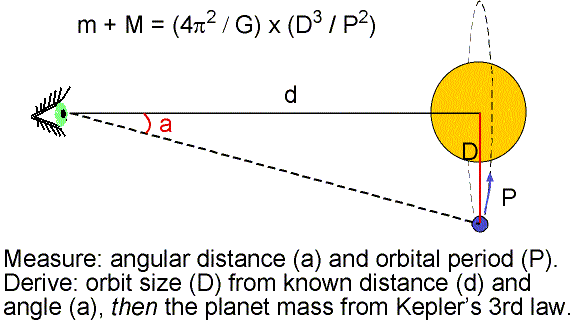Isaac Newton used his laws of motion and gravity to generalize Kepler's third law of planet orbits to cover any case where one object orbits another. He found for any two objects orbiting each other, the sum of their masses, planet mass + moon mass = (4p2/G) × [(their distance apart)3/(their orbital period around each other)2]. Newton's form of Kepler's third law can, therefore, be used to find the combined mass of the planet and the moon from measurements of the moon's orbital period and its distance from the planet.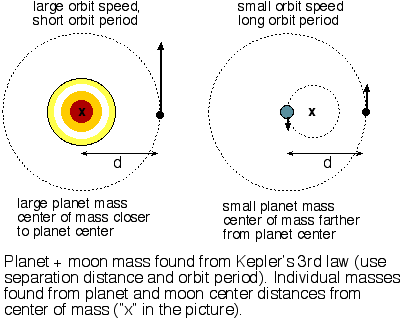You can usually ignore the mass of the moon compared to the mass of the planet because the moon is so much smaller than the planet, so Kepler's third law gives you the planet's mass directly. Examples are given in the Newton's Law of Gravity chapter. The one noticeable exception is Pluto and its moon, Charon. Charon is massive enough compared to Pluto that its mass cannot be ignored. The two bodies orbit around a common point that is proportionally closer to the more massive Pluto. The common point, called the center of mass, is 7.3 times closer to Pluto, so Pluto is 7.3 times more massive than Charon. Before the discovery of Charon in 1978, estimates for Pluto's mass ranged from 10% the Earth's mass to much greater than the Earth's mass. After Charon's discovery, astronomers found that Pluto is only 0.216% the Earth's mass---less massive than the Earth's Moon! For planets without moons (Mercury and Venus), you can measure their gravitational pull on other nearby planets to derive an approximate mass or, for more accurate results, measure how quickly spacecraft are accelerated when they pass close to the planets.

### Sizes and Volumes

The physical size of a planet can be found from measurements of its angular size and its distance. How large something appears to be is its angular size or angular diameter---the angle between two lines of sight along each side of the object. How big something appears to be obviously depends on its distance from us---it appears bigger when it is closer to us. Every time you drive a car or ride a bicycle, you use another car's or bicycle's angular size to judge how far away it is from you. You assume that you are not looking at some toy model. The planets are close enough to the Earth that you can see a round disk and, therefore, they have a measurable angular size. All of the stars (except the Sun) are so far away that they appear as mere points in even the largest telescopes, even though they are actually much larger than the planets.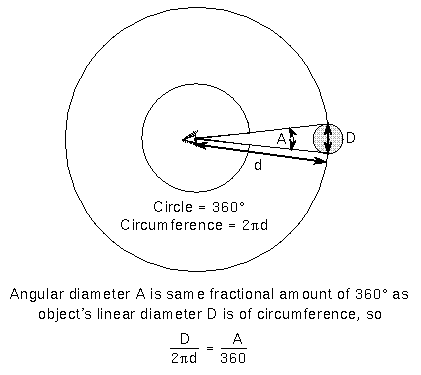If you know how far away a planet is from you, you can determine its linear diameter D. The diameter of a planet D = 2p × (distance to the planet) × (the planet's angular size in degrees)/360°, where the symbol p is a number approximately equal to 3.14 (your calculator may say 3.141592653...). The figure above explains where this formula comes from. This technique is used to find the actual diameters of other objects as well, like moons, star clusters, and even entire galaxies.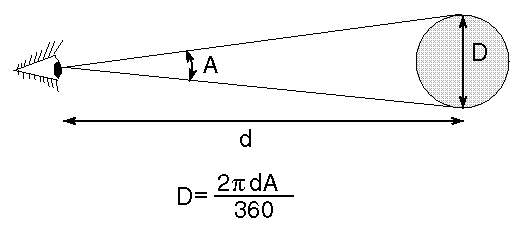### How do you do that?

As the planets orbit the Sun, their distance from us changes. At ``opposition'' (when they are in the direct opposite direction from the Sun in our sky) a planet gets closest to us. These are the best times to study a planet in detail. The planet Mars reaches opposition every 780 days. Because of their elliptical orbits around the Sun, some oppositions are more favorable than others. Every 15--17 years Mars is at a favorable opposition and approaches within 55 million kilometers to the Earth. At that time its angular size across its equator is 25.5 arc seconds. In degrees this is 25.5 arc seconds × (1 degree/3600 arc seconds) = 0.00708 degrees, cancelling out arc seconds top and bottom.

Its actual diameter = (2p × 55,000,000 km × 0.00708°)/360° = 6800 kilometers. Notice that you need to convert arc seconds to degrees to use the angular size formula.

Little Pluto is so small and far away that its angular diameter is very hard to measure. Only a large telescope above the Earth atmosphere (like the Hubble Space Telescope) can resolve its tiny disk. However, the discovery in 1978 of a moon, called Charon, orbiting Pluto gave another way to measure Pluto's diameter. Every 124 years, the orientation of Charon's orbit as seen from the Earth is almost edge-on, so you can see it pass in front of Pluto and then behind Pluto. This favorable orientation lasts about 5 years and, fortunately for us, it occurred from 1985 to 1990.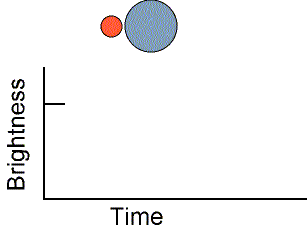When Pluto and Charon pass in front of each other, the total light from the Pluto-Charon system decreases. The length of time it takes for the eclipse to happen and the speed that Charon orbits Pluto can be used to calculate their linear diameters. Recall that the distance traveled = speed×(time it takes). Pluto's diameter is only about 2270 kilometers (about 65% the size of our Moon!) and Charon is about 1170 kilometers across. This eclipsing technique is also used to find the diameters of the very far away stars in a later chapter. Pluto's small size and low mass (see the previous section) have some astronomers calling it an ``overgrown comet'' instead of a planet and it was recently re-classified as a "dwarf planet".

Another way to specify a planet's size is to use how much space it occupies, i.e., its volume. Volume is important because it and the planet's composition determine how much heat energy a planet retains after its formation billions of years ago. Also, in order to find the important characteristic of density (see the next section), you must know the planet's volume.

Planets are nearly perfect spheres. Gravity compresses the planets to the most compact shape possible, a sphere, but the rapidly-spinning ones bulge slightly at the equator. This is because the inertia of a planet's material moves it away from the planet's rotation axis and this effect is strongest at the equator where the rotation is fastest (Jupiter and Saturn have easily noticeable equatorial bulges). Since planets are nearly perfect spheres, a planet's volume can be found from volume = (p/6) × diameter3. Notice that the diameter is cubed. Even though Jupiter has ``only'' 11 times the diameter of the Earth, over 1300 Earths could fit inside Jupiter! On the other end of the scale, little Pluto has a diameter of just a little more than 1/6th the diameter of the Earth, so almost 176 Plutos could fit inside the Earth.

### Densities and Compositions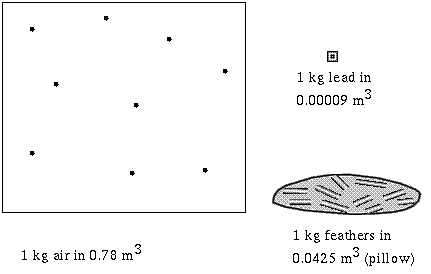An important property of a planet that tells what a planet is made of is its density. A planet's density is how much material it has in the space the planet occupies: density = mass/volume. Planets can have a wide range of sizes and masses but planets made of the same material will have the same density regardless of their size and mass. For example, a huge, massive planet can have the same density as a small, low-mass planet if they are made of the same material. I will specify the density relative to the density of pure water because it has an easy density to rememeber: 1 gram/centimeter3 or 1000 kilograms/meter3.

The four planets closest to the Sun (Mercury, Venus, Earth, Mars) are called the terrestrial planets because they are like the Earth: small rocky worlds with relatively thin atmospheres.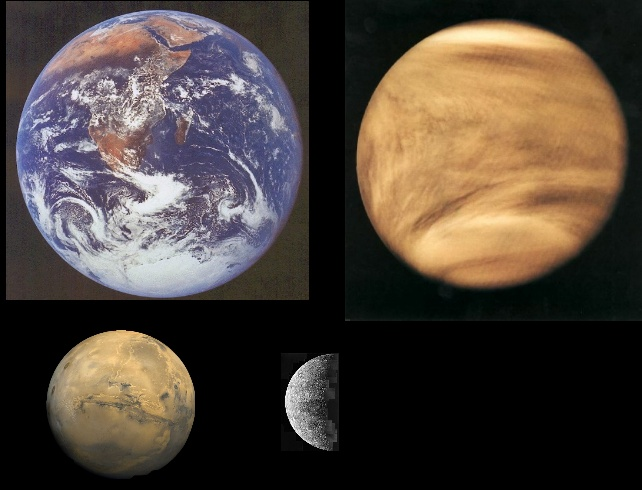The terrestrial planets to the same scale (using images from NASA and JPL). From top left and proceeding clockwise: Earth, Venus, Mercury, Mars (at bottom left).

Terrestrial (Earth-like) planets have overall densities = 4-5 (relative to the density of water) with silicate rocks on the surface. Silicate rock has density = 3 (less than the average density of a terrestrial planet) and iron has a density = 7.8 (more than the average density of a terrestrial planet). Since terrestrial planets have average densities greater than that for the silicate rocks on their surface, they must have denser material under the surface to make the overall average density what it is. Iron and nickel are present in meteorites (chunks of rock left over from the formation of the solar system) and the presence of magnetic fields in some of the terrestrial planets shows that they have cores of iron and nickel. Magnetic fields can be produced by the motion of liquid iron and nickel. Putting these facts together leads to the conclusion that the terrestrial planets are made of silicate rock surrounding a iron-nickel core.

The four giant planets beyond Mars (Jupiter, Saturn, Uranus, Neptune) are called the jovian planets because they are like Jupiter: large, mostly liquid worlds with thick atmospheres.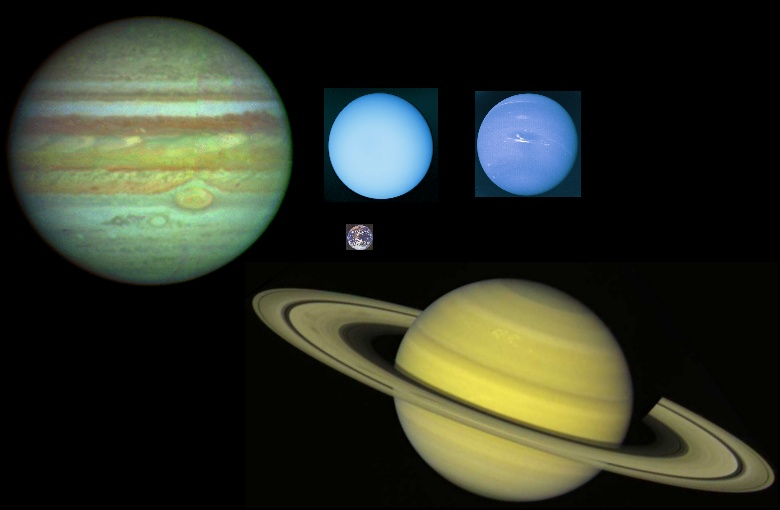The jovian planets to the same scale (using images from NASA and JPL). From top left and proceeding clockwise: Jupiter, Uranus, Neptune, Saturn (at bottom right). The Earth is also included to the same scale at center.

Jovian (Jupiter-like) planets have overall densities = 0.7-1.7 (relative to the density of water) with light gases visible on top. Gases and light liquids (like hydrogen and helium) have densities lower than water. Using reasoning similar to before you conclude that the jovian planets are made of gaseous and liquid hydrogen, helium and water surrounding a possible relatively small rocky core. Spectroscopy says the jovian planets have hydrogen, helium, methane, ammonia, and water gas in their thick atmospheres so the predictions are not too far off track.

The properties determined for each planet are given in the Planet Properties table. Clicking on the planet's name will bring up the full fact sheet for that planet. The important properties are given in the table.

### Vocabulary

angular diameter angular size center of mass
density

### Formulae

1. Planet mass = (4p2/G) × [distance3/(moon's orbital period)2] - moon's mass. The moon's mass can usually be ignored.
2. Planet diameter = 2p × (distance to the planet) × (planet's angular size in degrees)/360°.
3. Planet volume = (p/6) × (planet diameter)3.
4. Density = mass/volume.

### Review Questions

1. How does angular size depend on distance? (As its distance increases, does an object's angular size increase or decrease?)
2. How large is the Moon if its angular size is about 30 arc minutes and it is 384,000 kilometers away from us?
3. How many times biggger is the Sun than the Moon if the Sun is about 390 times farther away than the Moon but has the same angular size? What is the Sun's diameter in kilometers? (Hint: use the Moon data given in the previous question.)
4. If two objects at the same distance from us have different angular sizes with one 22 times smaller than the other, how many times larger in linear diameter is one than the other? If the large object is 141,000 kilometers across, how large is the other object?
5. A planet 140,000 kilometers across is 780 million kilometers from the Sun and rotates every 9.8 hours on its axis. It has a moon that orbits 380,000 km from the planet's center in 1.8 days. Explain what information you would use to find the mass of the planet and how the mass could be determined.
6. The Earth has a radius of 6400 kilometers and a mass of 6.0 × 1024 kilograms. What is its density?
7. A planet has a rocky surface of material with density 3 (relative to the density of water) and an interior of material with density 2.5. Will its overall average density be greater or less than 3? If the planet's interior is made of material with density 9, will the overall average density be greater or less than 3?

#### Go to Astronomy Notes home -- Please support this website

last updated: June 5, 2019